Do you like the article?
Share it with others -
post a link to it!
Use new possibilities of MetaTrader 5

# Fuzzy Logic in trading strategies

15 November 2017, 08:44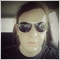21
25 522

### Introduction

Traders often wonder how to improve a trading system or create a new one through machine learning. Despite the abundance of publications, a simple and intuitive method is yet to be found for creating models that cannot be analytically estimated without resorting to computer-aided calculations. Fuzzy logic is a window to the world of machine learning. Combined with genetic algorithms, it is able to expand the capabilities of creating self-learning or easily optimizable trading systems. At the same time, fuzzy logic is intuitive, as it encapsulates crisp numerical information in fuzzy (blurred) terms, just like a person does in the process of thinking.

Here is an example. In terms of crisp logic, the speed of a moving car is determined by measurement devices: for instance, 60 km/h. But a casual observer with no measurement devices can only roughly estimate the speed of the car, relying on his experience or knowledge base. For instance, it is known that a car can go fast, and "fast" is defined approximately as 100 km/h and above. It is also known that a car can go slow, which is 5-10 km/h. And finally, the speed is visually estimated as average (around 60km/h) if the approaching car increases in size at a moderate rate. Thus, it is possible to characterize 60 km/h with four different expressions:

• average speed;
• speed close to average;
• more average than fast;
• and, finally, more average than slow.

This is how the information is encapsulated in a human consciousness, allowing him to grasp only the information necessary at the current moment, for example: "will I have time to run across the road if the car is moving not very fast?". Thinking about everything at once and in great detail would force a person to spend colossal amounts of time and energy resources before making any specific decision: run across the road or let the car pass. At the same time, the current situation would be thoroughly studied, which may never be identically repeated in the future, and would only have similar outlines. In machine learning, such situations are called overfitting.

This article will not delve into the fuzzy logic theory. Information on this topic is widely available on the Internet and on the MQL5 site. Let us start with the practice right away, which will be explained with theory excerpts and curious facts.

To construct a model, the Fuzzy library is used, available in the standard MetaTrader 5 terminal package.

The result will be a ready-made Expert Advisor based on fuzzy logic, which can be taken as an example for building custom systems.

### Creating a prototype of the trading system

Let us move on to creating crisp TS logic, which will be used as the foundation in further research. Then 2 identical systems can be compared, where the second one will utilize the fuzzy logic.

3 RSI oscillators with different periods will be used as the basis:

```hnd1 = iRSI(_Symbol,0,9,PRICE_CLOSE);
hnd2 = iRSI(_Symbol,0,14,PRICE_CLOSE);
hnd3 = iRSI(_Symbol,0,21,PRICE_CLOSE);
```

Let us formulate the crisp conditions of the signals and define them in the function:

```double CalculateSignal()
{
double res =0.5;
CopyBuffer(hnd1,0,0,1,arr1);
CopyBuffer(hnd2,0,0,1,arr2);
CopyBuffer(hnd3,0,0,1,arr3);

if(arr1>70 && arr2>70 && arr3>70) res=1.0;                    // if all indicators are in the overbought area, sell
if(arr1<30 && arr2<30 && arr3<30) res=0.0;                    // if all indicators are in the oversold area, buy

if(arr1<30 && arr2<30 && arr3>70) res=0.5;                    // if 2 are oversold, and 1 is overbought, no signal
if(arr1<30 && arr2>70 && arr3<30) res=0.5;
if(arr1>70 && arr2<30 && arr3<30) res=0.5;

if(arr1>70 && arr2>70 && arr3<30) res=0.5;                    // if 2 are overbought, and 1 is oversold, no signal
if(arr1>70 && arr2<30 && arr3>70) res=0.5;
if(arr1<30 && arr2>70 && arr3>70) res=0.5;

if(arr1<30 && arr2<30 && (arr3>40 && arr3<60)) res=0.0;    // if 2 are oversold, and 3rd is in the range of 40 to 60, the signal is to buy
if(arr1<30 && (arr2>40 && arr2<60) && arr3<30) res=0.0;
if((arr1>40 && arr1<60) && arr2<30 && arr3<30) res=0.0;

if(arr1>70 && arr2>70 && (arr3>40 && arr3<60)) res=1.0;    // if 2 are overbought, and 3rd is in the range of 40 to 60, the signal is to sell
if(arr1>70 && (arr2>40 && arr2<60) && arr3>70) res=1.0;
if((arr1>40 && arr1<60) && arr2>70 && arr3>70) res=1.0;

return(res);
}
```

Next, let us write all other service functions and test the expert from beginning of 2017 on EURUSD, timeframes М15 and М5 (the full code of the expert is attached at the end of the article):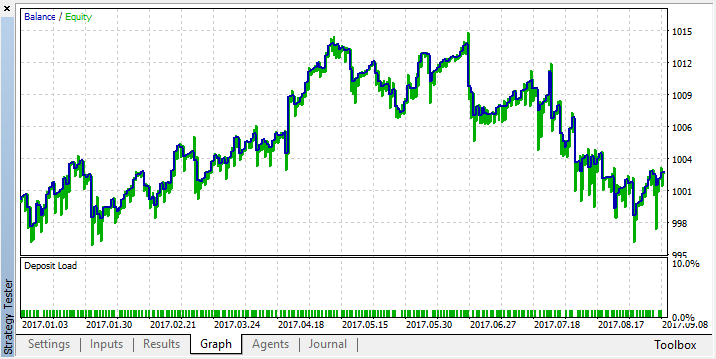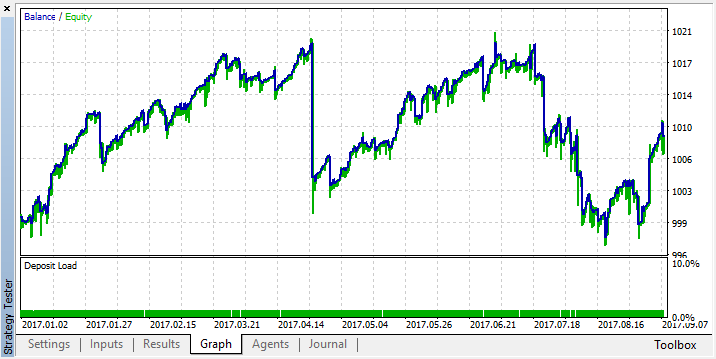Even though crisp conditions for combinations of the three indicators have been defined and the conditions are logical and consistent, this approach turned out to be too straightforward and inflexible. On average, the system neither loses nor earns for a period of 8 months. To make it earn, it would be necessary to go through a multitude of condition combinations, and possibly add more oscillators. But there is not much left to optimize, since the conditions are set extremely precisely.

Let us try to blur away the ideas about the conditions for making this trading system profitable using fuzzy logic.

### Creating a fuzzy logic model

First, it is necessary to include the Fuzzy library. To be exact, one of the two fuzzy logic models available - Mamdani or Sugeno. The difference between them is that Sugeno outputs a linear model without creating an output variable in the form of a fuzzy term set, whereas Mamdani provides this element. Since the article is written for fuzzy traders, Mamdani will be used. But this does not imply that the Sugeno model is unsuitable for some specific tasks: it is always possible and necessary to experiment relying on the basic understanding of fuzzy logic.

```#include <Math\Fuzzy\MamdaniFuzzySystem.mqh>
CMamdaniFuzzySystem *OurFuzzy=new CMamdaniFuzzySystem();
```

The library is included, a reference to the Mamdani class is declared. This is all that is needed to get started.

Now let us consider the main stages of constructing fuzzy inference. It occupies a central place in fuzzy modeling systems. The fuzzy inference process is a specific procedure or an algorithm for obtaining fuzzy conclusions based on fuzzy assumptions using the basic operations of fuzzy logic.

There are 7 stages of constructing fuzzy inference.

• Determining the structure of the fuzzy inference system.

The number of inputs and outputs, as well as the membership functions are defined at the design stage. In our case, there will be 4 inputs, 1 output, and each of them will have 3 membership functions.

• Forming the rule base of the fuzzy inference system.

During the development process, we create custom rules for fuzzy inference, based on our expert judgment of the trading system.

• Fuzzification of input variables.

Setting correspondence between the numerical value of the input variable of the fuzzy inference system and the value of the membership function of the corresponding term of the linguistic variable.

• Aggregation

The procedure of determining the degree of truth of conditions for each rule of the fuzzy inference system.

• Activation

The process of finding the truth degree of each of the elementary propositions (subclauses) constituting the consequents of kernels of all fuzzy production rules.

• Accumulation

The process of finding a membership function for each of the output linguistic variables.

• Defuzzification
The process of transition from the membership function of the output linguistic variable to its crisp (numerical) value. This will be the output value in the range from 0 to 1.

It should be noted that only the points 1 and 2 need to be performed, all others will be done by the system without intervention. Those interested in the subtleties of the fuzzy logic operation at all stages can find more details here.

### Determining the structure of the fuzzy inference system

Let us continue with the creation of the model. Define objects of three inputs and one output, as well as auxiliary objects of dictionary to facilitate the work with the logic:

```CFuzzyVariable *firstInput=new CFuzzyVariable("rsi1",0.0,1.0);
CFuzzyVariable *secondInput=new CFuzzyVariable("rsi2",0.0,1.0);
CFuzzyVariable *thirdInput=new CFuzzyVariable("rsi3",0.0,1.0);
CFuzzyVariable *fuzzyOut=new CFuzzyVariable("out",0.0,1.0);

CDictionary_Obj_Double *firstTerm=new CDictionary_Obj_Double;
CDictionary_Obj_Double *secondTerm=new CDictionary_Obj_Double;
CDictionary_Obj_Double *thirdTerm=new CDictionary_Obj_Double;
CDictionary_Obj_Double *Output;
```

Three RSI with different periods will be used as inputs. Since the RSI oscillator is always in the range of 0—100, it is necessary to create a variable for it with the same dimension. But for convenience, the indicator values will be normalized to a range of 0—1. Simply keep in mind that the created variable must have a dimension equal to the dimension of the input vector, i.e. it must hold all the values. A range from 0 to 1 is set at the output as well.

According to point 1 of fuzzy logic creation, it is also necessary to define and configure the membership functions. This will be done in the OnInit() event handler:

```firstInput.Terms().Add(new CFuzzyTerm("buy", new CZ_ShapedMembershipFunction(0.0,0.6)));
firstInput.Terms().Add(new CFuzzyTerm("neutral", new CNormalMembershipFunction(0.5, 0.2)));
firstInput.Terms().Add(new CFuzzyTerm("sell", new CS_ShapedMembershipFunction(0.4,1.0)));
OurFuzzy.Input().Add(firstInput);

secondInput.Terms().Add(new CFuzzyTerm("buy", new CZ_ShapedMembershipFunction(0.0,0.6)));
secondInput.Terms().Add(new CFuzzyTerm("neutral", new CNormalMembershipFunction(0.5, 0.2)));
secondInput.Terms().Add(new CFuzzyTerm("sell", new CS_ShapedMembershipFunction(0.4,1.0)));
OurFuzzy.Input().Add(secondInput);

thirdInput.Terms().Add(new CFuzzyTerm("buy", new CZ_ShapedMembershipFunction(0.0,0.6)));
thirdInput.Terms().Add(new CFuzzyTerm("neutral", new CNormalMembershipFunction(0.5, 0.2)));
thirdInput.Terms().Add(new CFuzzyTerm("sell", new CS_ShapedMembershipFunction(0.4,1.0)));
OurFuzzy.Input().Add(thirdInput);

fuzzyOut.Terms().Add(new CFuzzyTerm("buy", new CZ_ShapedMembershipFunction(0.0,0.6)));
fuzzyOut.Terms().Add(new CFuzzyTerm("neutral", new CNormalMembershipFunction(Gposition, Gsigma)));
fuzzyOut.Terms().Add(new CFuzzyTerm("sell", new CS_ShapedMembershipFunction(0.4,1.0)));
OurFuzzy.Output().Add(fuzzyOut);
```

Now let us see what the membership function is and what purpose it serves.

Three terms have been created for each input (and one output) variable: "buy", "neutral", "sell", each with its own membership function. In other words, the oscillator values can now be divided into 3 fuzzy groups, and each group can be assigned a range of values using the membership function. Speaking in the language of fuzzy logic, 4 term sets have been created, each of which has 3 terms. To illustrate the above, we will write a simple script that can be used for visualization of the terms and their membership functions:

```//+------------------------------------------------------------------+
//|                                      Our MembershipFunctions.mq5 |
//|                        Copyright 2016, MetaQuotes Software Corp. |
//|                                             https://www.mql5.com |
//+------------------------------------------------------------------+
#include <Math\Fuzzy\membershipfunction.mqh>
#include <Graphics\Graphic.mqh>
//--- Create membership functions
CZ_ShapedMembershipFunction func2(0.0, 0.6);
CNormalMembershipFunction func1(0.5, 0.2);
CS_ShapedMembershipFunction func3(0.4, 1.0);

//--- Create wrappers for membership functions
double NormalMembershipFunction1(double x) { return(func1.GetValue(x)); }
double ZShapedMembershipFunction(double x) { return(func2.GetValue(x)); }
double SShapedMembershipFunction(double x) { return(func3.GetValue(x)); }

//+------------------------------------------------------------------+
//| Script program start function                                    |
//+------------------------------------------------------------------+
void OnStart()
{
//--- create graphic
CGraphic graphic;
if(!graphic.Create(0,"Our MembershipFunctions",0,30,30,780,380))
{
graphic.Attach(0,"Our MembershipFunctions");
}
graphic.HistoryNameWidth(70);
graphic.BackgroundMain("Our MembershipFunctions");
graphic.BackgroundMainSize(16);
//--- create curve
graphic.CurveAdd(NormalMembershipFunction1,0.0,1.0,0.01,CURVE_LINES,"[0.5, 0.2]");
graphic.CurveAdd(ZShapedMembershipFunction,0.0,1.0,0.01,CURVE_LINES,"[0.0, 0.6]");
graphic.CurveAdd(SShapedMembershipFunction,0.0,1.0,0.01,CURVE_LINES,"[0.4, 1.0]");
//--- sets the X-axis properties
graphic.XAxis().AutoScale(false);
graphic.XAxis().Min(0.0);
graphic.XAxis().Max(1.0);
graphic.XAxis().DefaultStep(0.1);
//--- sets the Y-axis properties
graphic.YAxis().AutoScale(false);
graphic.YAxis().Min(0.0);
graphic.YAxis().Max(1.1);
graphic.YAxis().DefaultStep(0.1);
//--- plot
graphic.CurvePlotAll();
graphic.Update();
}
```

Run the script on the chart: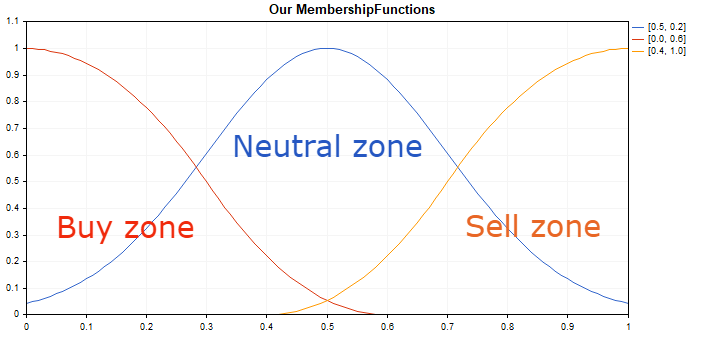These membership functions have been selected, because they have only 2 optimizable input parameters (this will be done later, during the system testing stage). They also describe the extreme and central positions of the system well. You can apply any membership function from the ones available in the Fuzzy library.

Let us adopt a rule that the extreme values of the oscillator indicate an upcoming change in its direction and, consequently, an upcoming trend reversal. Therefore, the oscillator approaching to zero hints at a possible beginning of growth. Movement of the oscillator to the 0.5 mark is accompanied by a gradual decrease in CZ_ShapedMembershipFunction or term "Buy zone". At the same time, the uncertainty in CNormalMembershipFunction of "Neutral zone" will grow, which is eventually replaced by an increase in CS_ShapedMembershipFunction or "Sell zone" as the oscillator approaches 1. The same principle is used in all inputs and output, which check if the indicator values belong to a particular zone with fuzzy boundaries.

There are no restrictions on the number of membership functions for each variable. You can set 5, 7, 15 functions instead of three, but, of course, within the limits of common sense and in the name of fuzzy logic.

### Forming the rule base of the fuzzy inference system.

At this stage, we add a knowledge base to the system to be used when making fuzzy decisions.

```   rule1 = OurFuzzy.ParseRule("if (rsi1 is buy) and (rsi2 is buy) and (rsi3 is buy) then (out is buy)");
rule2 = OurFuzzy.ParseRule("if (rsi1 is sell) and (rsi2 is sell) and (rsi3 is sell) then (out is sell)");
rule3 = OurFuzzy.ParseRule("if (rsi1 is neutral) and (rsi2 is neutral) and (rsi3 is neutral) then (out is neutral)");

rule4 = OurFuzzy.ParseRule("if (rsi1 is buy) and (rsi2 is sell) and (rsi3 is buy) then (out is neutral)");
rule5 = OurFuzzy.ParseRule("if (rsi1 is sell) and (rsi2 is sell) and (rsi3 is buy) then (out is neutral)");
rule6 = OurFuzzy.ParseRule("if (rsi1 is buy) and (rsi2 is buy) and (rsi3 is sell) then (out is neutral)");

rule7 = OurFuzzy.ParseRule("if (rsi1 is buy) and (rsi2 is buy) and (rsi3 is neutral) then (out is buy)");
rule8 = OurFuzzy.ParseRule("if (rsi1 is sell) and (rsi2 is sell) and (rsi3 is neutral) then (out is sell)");
rule9 = OurFuzzy.ParseRule("if (rsi1 is buy) and (rsi2 is neutral) and (rsi3 is buy) then (out is buy)");
rule10 = OurFuzzy.ParseRule("if (rsi1 is sell) and (rsi2 is neutral) and (rsi3 is sell) then (out is sell)");
rule11 = OurFuzzy.ParseRule("if (rsi1 is neutral) and (rsi2 is buy) and (rsi3 is buy) then (out is buy)");
rule12 = OurFuzzy.ParseRule("if (rsi1 is neutral) and (rsi2 is sell) and (rsi3 is sell) then (out is sell)");

OurFuzzy.Rules().Add(rule1);
OurFuzzy.Rules().Add(rule2);
OurFuzzy.Rules().Add(rule3);
OurFuzzy.Rules().Add(rule4);
OurFuzzy.Rules().Add(rule5);
OurFuzzy.Rules().Add(rule6);
OurFuzzy.Rules().Add(rule7);
OurFuzzy.Rules().Add(rule8);
OurFuzzy.Rules().Add(rule9);
OurFuzzy.Rules().Add(rule10);
OurFuzzy.Rules().Add(rule11);
OurFuzzy.Rules().Add(rule12);
```

At least one logical condition must be added to the knowledge base: it is considered incomplete if at least one term is not involved in logical operations. There can be an indefinite amount of logical conditions.

The provided example sets 12 logical conditions, which influence the fuzzy inference when met. Thus, all terms participate in logical operations. By default, all logical operations are assigned the same weight coefficients equal to 1. They will not be changed in this example.

If all 3 indicators are within the fuzzy area for buying, a fuzzy buy signal will be output. The same applies to sell and neutral signals. (rules 1-3)

If 2 indicators show buy and one shows sell, the output value will be neutral, that is, uncertain. (rules 4-6)

If 2 indicators show buy or sell, and one is neutral, then buy or sell is assigned to the output value. (rules 7-12)

Obviously, this is not the only variant for creating a rule base, you are free to experiment. This rule base is founded merely on my "expert" judgment and vision of how the system should function.

### Obtaining a crisp output value after defuzzification

It remains to calculate the model and obtain the result as a value from 0 to 1. Values close to 0 will indicate a strong buy signal, those close to 0.5 are neutral, and values close to 1 mean a strong sell signal.

```double CalculateMamdani()

{
CopyBuffer(hnd1,0,0,1,arr1);
NormalizeArrays(arr1);

CopyBuffer(hnd2,0,0,1,arr2);
NormalizeArrays(arr2);

CopyBuffer(hnd3,0,0,1,arr3);
NormalizeArrays(arr3);

firstTerm.SetAll(firstInput,arr1);
secondTerm.SetAll(secondInput,arr2);
thirdTerm.SetAll(thirdInput,arr2);

Inputs.Clear();
Inputs.Add(firstTerm);
Inputs.Add(secondTerm);
Inputs.Add(thirdTerm);

CList *FuzzResult=OurFuzzy.Calculate(Inputs);
Output=FuzzResult.GetNodeAtIndex(0);
double res = Output.Value();
delete FuzzResult;

return(res);
}
```

This function gets the values of three RSI oscillators with different periods, normalizes them to a range from 0 to 1 (values can be simply divided by 100), updates the list with objects of the Fuzzy dictionary (the latest indicator values), sends it to calculations, creates a list for the output variable and takes the result in the 'res' variable.

### Adding service functions and optimizing/testing the resulting system

Since machine learning or at least its basics are also being considered, some parameters will be moved to inputs and optimized.

```input string Fuzzy_Setings;        //Fuzzy optimization settings
input double Gsigma = 0.5;         //sigma From 0.05 to 0.5 with 0.05 step
input double Gposition=0.5;        //position From 0.0 to 1.0 with 0.1 step
input double MinNeutralSignal=0.4; //MinNeutralSignal from 0.3 to 0.5 with 0.1 step
input double MaxNeutralSignal=0.6; //MaxNeutralSignal from 0.5 to 0.7 with 0.1 step
```

The parameters of the Gaussian (membership function) will undergo optimization at the output of the fuzzy logic. It will have its center along the X axis shifted (parameter Gposition), its sigma changed (its bell narrowed and compressed, parameter Gsigma). This will give a better fine-tuning of the system in case the RSI signals for buying and selling are asymmetric.

Additionally, optimize the conditions for opening deals: the minimum value of a neutral signal and the maximum value (new positions will not be opened in the range between these values, as the signal is not defined).

The processing of a signal at the output of the fuzzy logic is shown in the following listing:

```void OnTick()
{
//---
if(!isNewBar())
{
return;
}

double TradeSignal=CalculateMamdani();

if(CountOrders(0)!=0 || CountOrders(1)!=0)                                           // if there are open positions
{
for(int b=OrdersTotal()-1; b>=0; b--)
{
if(OrderSelect(b,SELECT_BY_POS)==true)
{
if(OrderSymbol()==_Symbol && OrderMagicNumber()==OrderMagic)
{
if(OrderType()==OP_BUY && TradeSignal>=MinNeutralSignal)                  // a buy order is selected and the trade signal is greater than the left boundary of the neutral signal
{                                                                        // that is, there is either a neutral signal or a sell signal
if(OrderClose(OrderTicket(),OrderLots(),OrderClosePrice(),0,Red))       // then close the buy position
{
if(TradeSignal>MaxNeutralSignal)                     // if the order is closed and a sell signal exists (exceeds the right boundary of the neutral signal), immediately open a sell position
{
lots = LotsOptimized();
if(OrderSend(Symbol(),OP_SELL,lots,SymbolInfoDouble(_Symbol,SYMBOL_BID),0,0,0,NULL,OrderMagic,Red)){
};
}
}
}
if(OrderType()==OP_SELL && TradeSignal<=MaxNeutralSignal)                 // a sell order is selected....///.... the same as for buy positions, but all conditions are mirrored
{
if(OrderClose(OrderTicket(),OrderLots(),OrderClosePrice(),0,Red))
{
if(TradeSignal<MinNeutralSignal)
{
lots = LotsOptimized();
if(OrderSend(Symbol(),OP_BUY,lots,SymbolInfoDouble(_Symbol,SYMBOL_ASK),0,0,0,NULL,OrderMagic,Green)){
};
}
}
}
}
}
}
return;
}
// if there are no positions, open positions according to signals
lots = LotsOptimized();
if(TradeSignal<MinNeutralSignal && CheckMoneyForTrade(_Symbol,lots,ORDER_TYPE_BUY))
{
if(OrderSend(Symbol(),OP_BUY,lots,SymbolInfoDouble(_Symbol,SYMBOL_ASK),0,0,0,NULL,OrderMagic,Green)){
};
}
else if(TradeSignal>MaxNeutralSignal && CheckMoneyForTrade(_Symbol,lots,ORDER_TYPE_SELL))
{
if(OrderSend(Symbol(),OP_SELL,lots,SymbolInfoDouble(_Symbol,SYMBOL_BID),0,0,0,NULL,OrderMagic,Red)){
};
}
return;

}
```

Calculations will be carried out on new bar to accelerate the demonstration. You are free to customize the logic at your discretion, for example, trade on every tick by simply removing the check for a new bar.

If there are open positions and the signal contradicts the current position or is not defined, close the position. If there is a condition for opening an opposite position, open it.

This system does not utilize stop loss, as it is not trade reversals, and closure/reopening of trades is based on signals.

The Expert Advisor uses the MT4Orders library to facilitate the work with orders and to make the code easily convertible into MQL4.

### Testing process

The system is optimized using the settings shown in the screenshots, on the M15 timeframe, on the period of 8 months, based on Open prices, using the fast genetic based algorithm and the Balance + max Profit optimization criterion.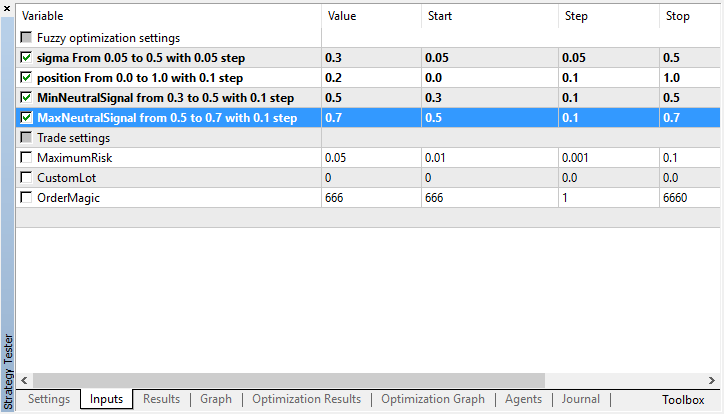Select the best optimization result: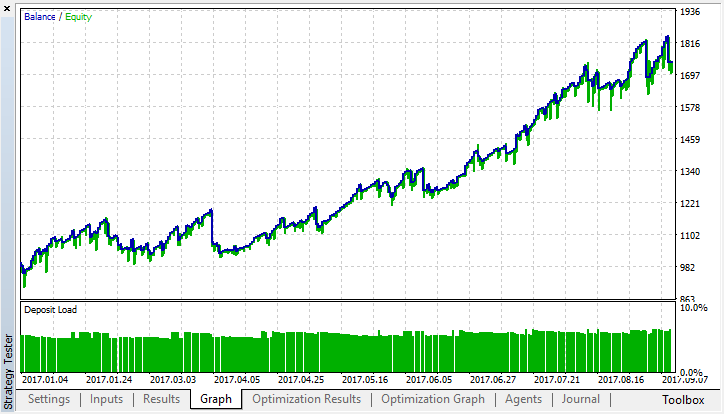Compare it with the testing results of the strict model:The resulting membership functions at the output, after optimization (the inputs remain unchanged since they were not optimized):

Before the changes:After: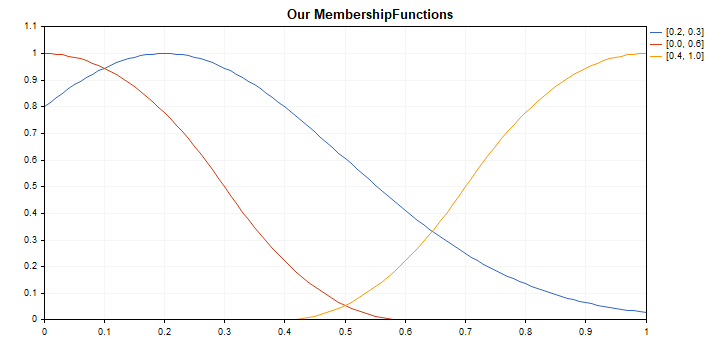Optimize the system with the same settings but on the M5 timeframe: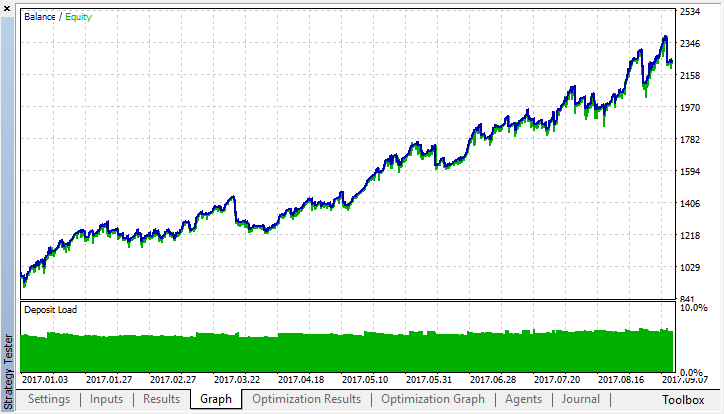Compare it with the testing results of the strict model:The resulting membership functions at the output, after optimization (the inputs remain unchanged since they were not optimized):

Before the changes:After: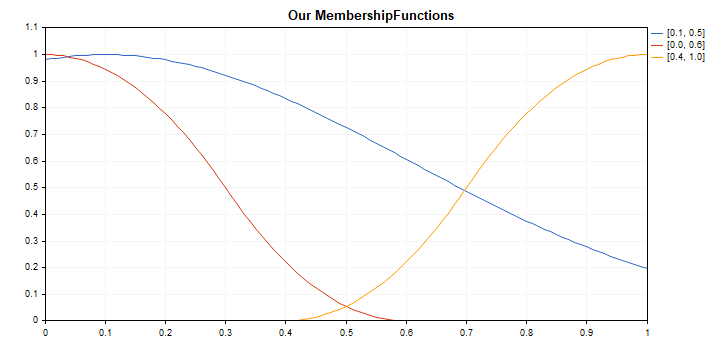In both cases, the Gaussian (neutral zone) was shifted towards buys and the number of long positions prevails over the number of short positions. This means that the buy and sell signals turned out to be asymmetrical on this particular segment of history, which could not be discovered without such an experiment. It is possible that the system consisting of three RSI was in the oversold zone (area 1) more often than in the overbought zone (area 0), and optimization of the Gaussian helped smooth out this imbalance. As for the crispest output, it is analytically hard to imagine why such an output configuration contributed to the improvement of the trading system results, because the process of defuzzification using the center of gravity method, in conjunction with all the mapping of inputs to fuzzy sets, is already a complex system by itself.

The system proved to be quite stable for 8 months, even though only 4 parameters were optimized. And they can easily be reduced to two (Gsigma and Gposition), since the remaining 2 had little impact on the result and are always in the vicinity of 0.5. This is assumed a satisfactory result for an experimental system, aimed at showing how the number of optimized parameters can be reduced by introducing an element of fuzzy logic into the trading system. In contrast, it would have been necessary to create numerous optimization criteria for strict rules, which would increase the complexity of system development and the number of optimized parameters.

It should also be noted that this is still a very crude example of building a trading system based on fuzzy logic, as it uses a primitive RSI-based strategy without even using stop losses. However, this should be sufficient to understand the applicability of fuzzy logic to creation of trading systems.

### Conclusion

Fuzzy logic allows for a quick creation of systems with fuzzy rules that are very simple to optimize. At the same time, the complex process of selecting the trading system parameters passes through genetic optimization, freeing the developer from the routine of searching for a trading strategy, developing and algorithmizing numerous rules of the trading system. Together with other machine learning methods (for example, neural networks), this approach allows achieving impressive results. It reduces the chance of overfitting and the dimension of the input data (3 RSI indicators with different periods narrowed down to a single signal, which describes the market situation more fully and more generalized than each indicator on its own).

If you still have troubles understanding how the fuzzy logic works, ask yourself how you think, what terms you operate and what rule bases your decision-making relies on.

Here is a reinforcement example. For instance, you have 3 wishes: go to a party, watch a movie at home or save the world. The term "watch a movie at home" has the greatest weight, because you are already at home and no further effort is necessary. Going to a party is viable if someone invites you and picks you up, but since it had not happened yet, the chances of going are average. And, finally, to save the world, you need to mobilize all your supernatural abilities, put on a superman costume and fight an alien monster. It is unlikely that you decide to do it today and not leave it until tomorrow, so the chances are slim.

The fuzzy inference will be something like this: I will most likely stay at home, and perhaps I will go to the party, but I am definitely not going to save the world today. After defuzzification, our chances could be evaluated on a scale of 0 to 10, where 0 is "stay at home", 5 is "go to the party", 10 is "fight a monster". Obviously, the crisp output would lie in the range of 0 to 3, i.e. you are most likely to stay at home. The same principle is used in the presented trading system: it compares the values of three indicators and uses logical conditions to determine the most preferable option at the current moment — buying, selling or doing nothing.

Possible ways to improve this example (for self-study):

• Increasing the number of inputs and logical conditions. This increases the capacity of the system and makes it more adaptive to the market.
• Optimizing not only the output Gaussian, but also all membership functions of inputs and output.
• Optimizing the rule base.
• Optimization the weights of logical expressions.
• Creating a committee of several fuzzy models responsible for different aspects of the trading system.
• Using fuzzy inferences as predictors ("features") and/or target variables for neural networks.

If there is enough interest in the article, and I receive sufficient feedback, I could consider the possibility of writing a new article devoted to combination of fuzzy logic and a neural network.

Below are the source codes of the experts and a test script for the membership functions. For the expert to compile and work, it is necessary to download the MT4Orders library and the updated Fuzzy library.

Translated from Russian by MetaQuotes Software Corp.
Original article: https://www.mql5.com/ru/articles/3795

#### Other articles by this author

Last comments | Go to discussion (21)| 10 Sep 2018 at 14:29
205836:

Hi, Thanks for your excellent article, Do you have any plan for writing an article about neural network(Boltzman, kohonen and) in mql5 ?

best regards

Hi, you can read here https://www.mql5.com/en/articles/3856

also new article soon maybe, maybe not..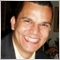| 18 Oct 2018 at 23:33

Hello,

This article has 3 terms: "buy", "sell" and "neutral".

when run Output.Value() we get a double value that correspond to Fuzzy result.

How I can convert this value to corresponding text term? or how I can get the text that correspond to the Fuzzy result?

I need the result like "buy" or "sell" or "neutral"

Thanks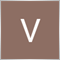| 7 Feb 2019 at 09:04

I'm pretty new to Trading, specifically Algo Trading. It is simply the ease with which I can read and make sense of the concepts you have explained.

I'm really interested in AI, Decision Trees, NN, etc. (well, I'm doing a course by Dr. E.P Chan and Quantra for that). This article will surely put me ahead in the race.

Thanks once again!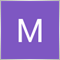| 25 Feb 2020 at 16:02
Maxim Dmitrievsky:

Hi, you can read here https://www.mql5.com/en/articles/3856

also new article soon maybe, maybe not..

hi maxim

thank for your fantastic samples

when i debug this ea

mql return error

incorect casting of pointers on RuleParser.mqh line 712

please help to fix this bug

my compiler version is

Version 5 build 2340 21 Feb 2020

many thanks to you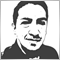| 15 Mar 2021 at 23:25
This is amazing... I implemented it with some small additions (like complementing the rules as suggested by author) in a triple channel' mean reversion strategy and improved its performance to the moon... thank you for sharing.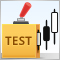Mini Market Emulator or Manual Strategy Tester
Mini Market Emulator is an indicator designed for partial emulation of work in the terminal. Presumably, it can be used to test "manual" strategies of market analysis and trading.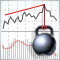A New Approach to Interpreting Classic and Hidden Divergence
The article considers the classic method for divergence construction and provides an additional divergence interpretation method. A trading strategy was developed based on this new interpretation method. This strategy is also described in the article.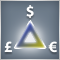Triangular arbitrage
The article deals with the popular trading method - triangular arbitrage. Here we analyze the topic in as much detail as possible, consider the positive and negative aspects of the strategy and develop the ready-made Expert Advisor code.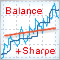Optimizing a strategy using balance graph and comparing results with "Balance + max Sharpe Ratio" criterion
In this article, we consider yet another custom trading strategy optimization criterion based on the balance graph analysis. The linear regression is calculated using the function from the ALGLIB library.